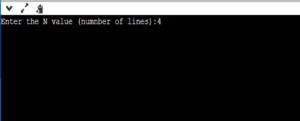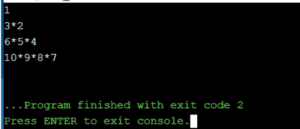# Print Number Star Right Triangle Pattern Type4

PRINT PATTERN  1 3*2 6*5*4 10*9*8*7

For any input number N Print the following code – For below code N=4

```1
3*2
6*5*4
10*9*8*7```

Starting from 1, each line contains ‘n’ number of digits where ‘n’ equals the line number and the digits occur in reverse chronological order with a (*) in between each number (Remember there is no (*) at the end of each line).

```1
3*2
6*5*4
10*9*8*7
15*14*13*12*11```

PREREQUISITE:-

Basic knowledge in C programming, usage of loops.

ALGORITHM:-

1. Take input from user i.e number of lines required (N value).
2. Take a result variable (say ‘a’) and initialize it with 1.
3. Take two loops one for each line (say ‘i’) and other for each digit in a particular line (say ‘j’).
4. Here ‘i’ loop is used to access each line from 1 to N and ‘j’ loop is used to print values in each line. For example in line 2 the value of i=2 and for contents in second line (i.e 3*2) the value for j for digit 3 is j=1 and for digit 2 is j=2.
5. Increment the ‘a’ value at the beginning of each line to N values by using (i*(i+1))/2 since we need to print digits in reverse order.
6. For example, When control is in line 2, since i=2, the corresponding ‘a’ value in the loop becomes (2*(2+1))/2=3. Hence 3* is printed for j=1 and is decremented since we used post decrement and for j=2 the digit 2 is printed.
7. Repeat the ‘i’ loop until it reaches ‘N’ lines.

CODE IN C:-

[code language=”cpp”]
#include<stdio.h>           /*Importing standard stdio.h library in order to use printf(), scanf() etc */
#include<conio.h>           /*Importing standard conio. library in order to use clrscr() etc */
void main()                 /* Beginning of the code (main block)*/

{

int N,i,j,a=1;        /*Integer variables declared N for number of lines. i, j, for loops, a for answer.*/
clrscr();               /*clears the screen */

printf("Enter the N value (number of lines):");
/* Indicating the user to give N value */

scanf("%d",&N);          /*receiving the input from user using scanf() */

for(i=1;i<=N;i++) {     /*Starting of i loop indicating number of lines in the output from 1 to N*/

a=(i*(i+1))/2;              /*skipping the value of a by N value, as the digits need to be printed in -reverse*/

for(j=1;j<i;j++) {      /*starting of j loop for printing digits in a particular line*/

printf("%d*",a–);     /*printing the value in a(except the last digit in each line  with * and post- decrementing it,*/

}

printf("%d\n",a–);   /*Printing the last digit in each line without * and going to next line */

}
}
[/code]

Output – 1 (Taking input from user):-Output – 2 (Displaying Result):-